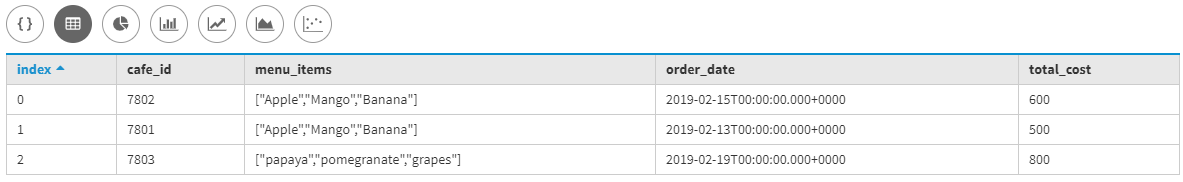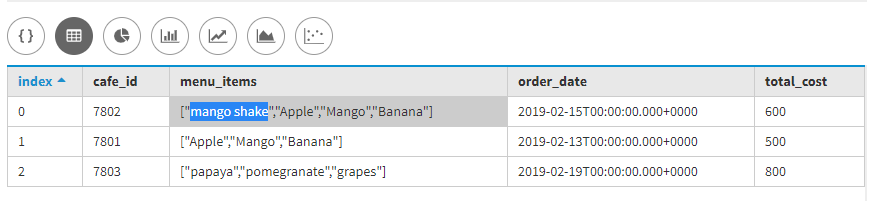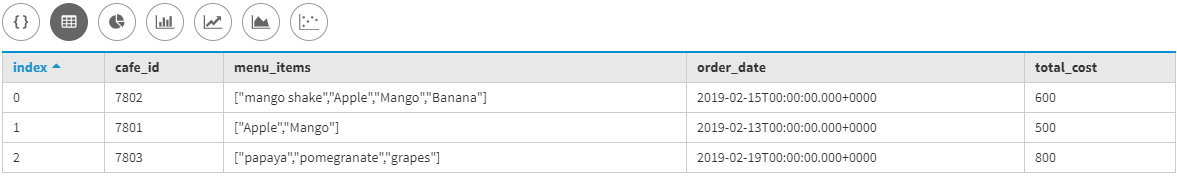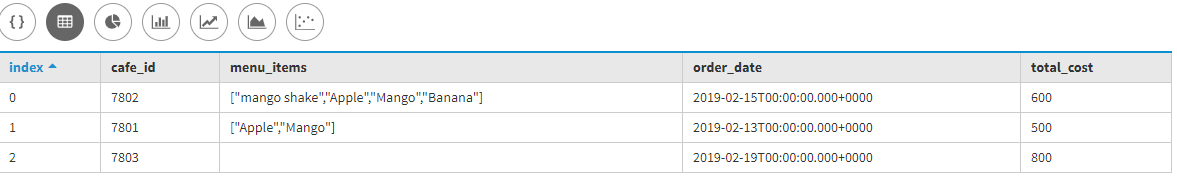Related Articles

# Updating Set in Cassandra

• Last Updated : 12 Dec, 2019

In this article, we will discuss how we can update set Collection Data Type in different ways also we will discuss how we can insert rows by using the UPDATE clause and if we don’t want any set elements then we can also remove using UPDATE clause with (–) operator.

First, we will create table Food_menu in which Café_id, Order_Date, total_cost, Menu_items are the fields in the below-given table. Let’s have a look.

```Create Table Food_menu
(
Cafe_id int Primary Key,
Order_Date Date,
total_cost int,
);```

Now, we are going to insert some data into the Food_menu table by using the following Cassandra Query Language (CQL) query given below. Let’s have a look.

```INSERT INTO Food_menu (Cafe_id,  Order_Date, total_cost, Menu_items)
VALUES (7801, '2019-02-13', 500, {'Banana', 'Mango', 'Apple'});

VALUES (7802, '2019-02-15', 600, {'Banana', 'Mango', 'Apple'});

VALUES (7803, '2019-02-19', 800, {'grapes', 'papaya', 'pomegranate'}); ```

Let’s see the output of the inserted data.

```select *

Output:Now, here we will discuss the updating the set collection data type. Let’s have a look.

1. We can add an element in set collection data type by using (+) operator.
```UPDATE Food_menu
WHERE Cafe_id = 7802; ```

Let’s see the output of the above CQL query.

```select *

Output:2. We can remove an element from a set by using subtraction (-) operator.
```UPDATE Food_menu
WHERE Cafe_id = 7801; ```

Let’s see the output of the following above CQL query.

```select *

Output:3. Now, if we want to remove all elements from a set then used the following CQL query given below.
```UPDATE Food_menu
```select *